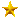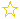VIP第11营业执照房源信息

VIP第13营业执照房源信息

VIP第1营业执照房源信息

VIP第1营业执照房源信息

VIP第7营业执照房源信息

• 出租
• [振嘉地产]
• 出租
• [振嘉地产]
• 出租
• [振嘉地产]
• 出租
• [振嘉地产]
• 出售
• [振嘉地产]
• 出租
• [振嘉地产]
• 出租
• [振嘉地产]
• 出租
• [振嘉地产]
• 出售
• [振嘉地产]
• 出租
• [振嘉地产]
• 出租
• [三缘商业地产]
• 出租
• [三缘商业地产]
• 出租
• [三缘商业地产]
• 出租
• [三缘商业地产]
• 出租
• [三缘商业地产]
• 出租
• [三缘商业地产]
• 出租
• [三缘商业地产]
• 出售
• [三缘商业地产]
• 出租
• [三缘商业地产]
• 出售
• [三缘商业地产]
• 出售
• [三缘商业地产]
• 出租
• [三缘商业地产]
• 出售
• [三缘商业地产]
• 出租
• [三缘商业地产]
• 出租
• [三缘商业地产]
• 出售
• [三缘商业地产]
• 出租
• [三缘商业地产]
• 出租
• [三缘商业地产]
• 出租
• [三缘商业地产]
• 出租
• [三缘商业地产]
• 出租
• [三缘商业地产]
• 出租
• [三缘商业地产]
• 出租
• [三缘商业地产]
• 出租
• [三缘商业地产]
• 出租
• [三缘商业地产]
• 出租
• [三缘商业地产]
• 出租
• [三缘商业地产]
• 出售
• [振嘉地产]
• 出租
• [振嘉地产]
• 出售
• [振嘉地产]
• 出租
• [振嘉地产]
• 出租
• [振嘉地产]
• 出售
• [振嘉地产]
• 出售
• [振嘉地产]
• 出售
• [振嘉地产]
• 出租
• [轩耀企服]
• 出租
• [轩耀企服]
• 出售
• [嘉腾地产]
• 出售
• [轩耀企服]
• 出租
• [轩耀企服]
• 出租
• [轩耀企服]
• 出售
• [嘉腾地产]
• 出租
• [轩耀企服]
• 出售
• [嘉腾地产]
• 出售
• [左邻右舍]
• 出售
• [嘉腾地产]
• 出租
• [嘉腾地产]
• 出售
• [嘉腾地产]
• 出租
• [左邻右舍]
• 出售
• [嘉腾地产]
• 出售
• [左邻右舍]
• 出售
• [嘉腾地产]
• 出售
• [左邻右舍]
• 出租
• [左邻右舍]
• 出售
• [轩耀企服]
• 出售
• [轩耀企服]
• 出租
• [轩耀企服]
• 出售
• [轩耀企服]
• 出租
• [轩耀企服]
• 出售
• [轩耀企服]
• 出租
• [轩耀企服]
• 出租
• [轩耀企服]
• 出租
• [轩耀企服]
• 出租
• [轩耀企服]
• 出租
• [轩耀企服]
• 出租
• [轩耀企服]
• 出租
• [轩耀企服]
• 出租
• [轩耀企服]
• 出租
• [轩耀企服]
• 出售
• [轩耀企服]
• 出租
• [左邻右舍]
• 出租
• [左邻右舍]
• 出售
• [嘉腾地产]
• 出售
• [嘉腾地产]
• 出售
• [嘉腾地产]
• 出售
• [嘉腾地产]
• 出售
• [嘉腾地产]
• 出售
• [嘉腾地产]
• 出售
• [嘉腾地产]
• 出售
• [嘉腾地产]
• 出售
• [轩耀企服]
• 出租
• [轩耀企服]
• 出租
• [轩耀企服]
• 出租
• [轩耀企服]
• 出租
• [轩耀企服]
• 出租
• [左邻右舍]
• 转让
• [左邻右舍]
• 出租
• [左邻右舍]